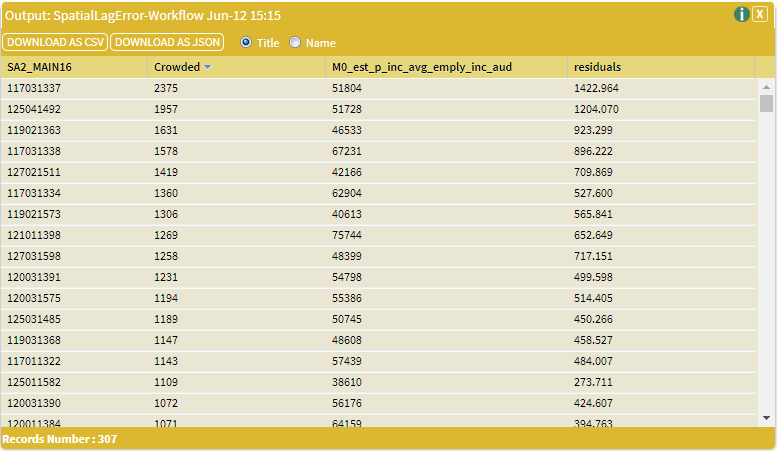# Spatial Lag Error Model (General Model)

Spatial Lag Error Model (General Model) combines the Spatial Lag and the Spatial Error regression models, giving us a spatially lagged dependent variable as well as a spatially lagged error term:

$\LARGE{y = \rho W_{1}y + X\beta + \mu}$

$\LARGE{\mu = \lambda W_{2}\mu + \epsilon}$

$\LARGE{\epsilon \sim N(0,\sigma^2 I)}$

Where $y$ is the n x 1 dependent variable vector (n being sample size), $\rho$ is the coefficient of the spatially lagged dependent variable, $W_{1}$ and $W_{2}$ are n x n spatial weight matrices ($W_{1} = W_{2}$ or $W_{1} \neq W_{2}$),

$X$ the n x k matrix of independent variables (k being the number of independent variables), $\beta$ the k x 1 vector of parameter estimates associated with the independent variables,

$\mu$ is the disturbance term, which has its own spatial autoregressive structure, where $\lambda$ is the coefficient of the spatially lagged error term and $\epsilon$ is the error term which is normally distributed with error variance matrix $\sigma^2 I_{n}$.

This model is sometimes referred to as the Kelejian-Prucha model, as they advocate this model throughout their work (Kelejian & Prucha, 1998).

### SET UP

To demonstrate this tool, we will explore household overcrowding and personal income within Greater Sydney.

Select Greater Sydney GCCSA as your area.

Select UNSW CFRC – Overcrowded Households Australia (SA2) 2016 as your dataset, with Total number of overcrowded households (Crowded) as the variable.

Select ABS – Data by Region – Income (Including Government Allowances) (SA2) 2011-2018 as your dataset, with the filter Year as 2016 and Estimates Of Personal Income Mean Employee Income $as the variable. Use the Merge Aggregated Datasets tool to Join the datasets. Use the Spatialise Aggregated Dataset tool to Spatialise the merged dataset. Use the Contiguous Spatial Weight Matrix tool to build a Spatial Weights Matrix for the spatialised dataset, using 1st order, row-standardised, Queen contiguity. ### Inputs Open the Spatial Lag Error Model (General Model) tool (Tools → Spatial Regression → Spatial Lag Error Model (General Model) and enter the following parameters: • Dataset Input: The dataset that contains the variable to be tested. Select the Spatialised Dataset. • Spatial Weights Matrix 01: The first spatial weight matrix to be used. Select the Contiguous Spatial Weight Matrix. • Spatial Weights Matrix 02: The second spatial weight matrix to be used. Select the Contiguous Spatial Weight Matrix. • Key Column: Specify the unique codes for your areas. Select SA2_MAIN16. • Dependent Variable: The dependent variable of the regression model. Select Total number of overcrowded households. • Independent Variable: The independent variable(s) of the regression model. Select Estimates Of Personal Income Mean Employee Income$.

The input parameters are summarised in the image below, once complete click Run Tool.### Outputs

Once the tool has finished, tick both boxes and click Display Output. This will open up two outputs.

The first output is a data file that you can map, containing your input variables and the following:

• Residuals: the residuals (difference between observed value and the fitted value) for each dependent variable in the regression model.The second output is a text window with a matrix of estimated parameters in the model:

• Regression Coefficients:
• Estimate: The estimate for the regression coefficients.
• Std. Error: The regression coefficient standard error.
• z value: The regression coefficient z-value.
• Pr(>|z|): The regression coefficient p-value.
• Rho:
• Estimate: The estimate for $\rho$.
• rho s.e.: The $\rho$ estimate standard error.
• z value: The $\rho$ estimate z-value.
• Pr(>|z|): The $\rho$ estimate p-value.
• Lambda:
• Estimate: The estimate for $\lambda$.
• lambda s.e.: The $\lambda$ estimate standard error.
• z value: The $\lambda$ estimate z-value.
• Pr(>|z|): The $\lambda$ estimate p-value.
• s2: The residual variance ($\sigma^2$).
• sigma: The Standard Deviation ($\sigma$).
• LL: The Log Likelihood (LL) value at computed optimum.
• AIC: The Akaike Information Criterion (AIC) of the linear model.
• LR: Likelihood (LR) ratio test result.
• Coefficient Covariance Matrix: Asymptotic coefficient covariance matrix for $\sigma$, $\rho$, $\lambda$ and the intercept.The output text is better viewed by copying or importing the data into a spreadsheet application.

Kelejian, H. H. & Prucha, I. R. (1998). A generalized spatial two-stage least squares procedure for estimating a spatial autoregressive model with autoregressive disturbances.
Journal of Real Estate Finance and Economics. 17(1), 99-121.

### Looking for Spatial Data?

You can browse the AURIN Data Discovery:### How can you Create Impact?